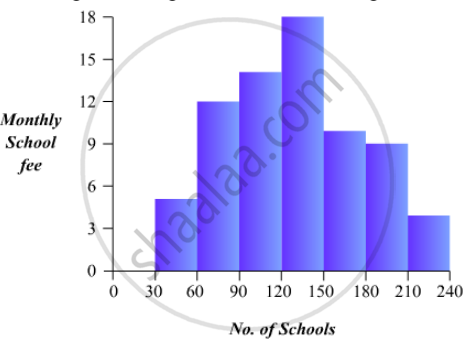# Construct a Histogram for the Following Data: Monthly School Fee (In Rs): 30-60 60-90 90-120 120-150 150-180 180-210 210-240 No of Schools 5 12 14 18 10 9 4 - Mathematics

Construct a histogram for the following data:

 Monthly Schoolfee (in Rs): 30-60 60-90 90-120 120-150 150-180 180-210 210-240 No of Schools 5 12 14 18 10 9 4

#### Solution

To represent the given data by a histogram, we first draw horizontal and vertical axes. Let us consider that the horizontal and vertical axes represent the class-limits and the frequencies of the class-intervals respectively.

The given data is a continuous grouped frequency distribution with equal class-intervals. Construct rectangles with class-intervals as bases and respective frequencies as heights. It should be noted that the scale for horizontal axis may not be same as the scale for vertical axis. Let us take one vertical division is equal to 3 rupees.

The heights of the different rectangles are as following

1. The height of the rectangle corresponding to the class-interval 30-60 is 5/3=1.66 big divisions.

2. The height of the rectangle corresponding to the class-interval 60-90 is 12/3 = 4 big divisions.

3. The height of the rectangle corresponding to the class-interval 90-120 is  14/3 = 4.66  big divisions.

4. The height of the rectangle corresponding to the class-interval 120-150 is 18/3=6 big divisions.

5. The height of the rectangle corresponding to the class-interval 150-180 is  10/30= 3.33  big divisions.

6. The height of the rectangle corresponding to the class-interval 180-210 is  9/3 = 3 big divisions.

7. The height of the rectangle corresponding to the class-interval 210-240 is 4/3 = 1.33 big divisions.

The histogram of the given data is the following:Concept: Graphical Representation of Data
Is there an error in this question or solution?

#### APPEARS IN

RD Sharma Mathematics for Class 9
Chapter 23 Graphical Representation of Statistical Data
Exercise 23.3 | Q 1 | Page 41

Share